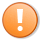# Quick-tip 50 | Find the lowest RSI and how many bars ago it occurred

Quick-tip 50 is an indicator is designed to look back a number of bars specified by user input: RSIMaxBarsBack, to find what the lowest value of RSI was (RSIMinValue) and how many bars ago it occurred (RSIMinBarsBack). The indicator plots the RSI value, RSIMinValue, and RSIMinBarsBack. It works on charts and RadarScreen.

Because the calculation of RSI is an accumulative calculation it is important to load sufficient data. On the chart this is done by setting how much history to load. In RadarScreen it is achieved by using the “Load additional data for accumulative calculations” setting. For the RSI calculation, TradeStation recommends a smoothing factor of 9.5 multiplied by the RSI length, in this case the user input RSILength.

Make sure that the chart is set to local time and the indicator is set to update value intra-bar.This content is for members only.